## Before you start…

Before starting this exercise, you should have completed all the previous Absolute Beginners’ workshop exercises. Each section below indicates which of the earlier worksheets are particularly relevant.

## Getting the data into R

Relevant worksheet: Introduction to RStudio

You’ll be provided with a CSV file before or during the workshop. If you want to try out this worksheet without that data file, you can use this example CSV file instead. You can only gain marks for this exercise if you use the CSV file from the workshop.

Create or open an appropriate project on RStudio Server for this analysis (Plymouth University students: use the project ‘psyc414’ created in the inter-rater reliability worksheet), upload your CSV to your project, and create a new R script called `corr.R`.

Relevant worksheet: Exploring data

``````library(tidyverse)

Note: In the example above, you’ll need to replace `corr.csv` with the name of the CSV file you just copied into your RStudio project.

### Inspect

Look at the data by clicking on it in the Environment tab in RStudio. Each row is one participant in one group. Here’s what each of the columns in the data set contain:

Column Description Values
SRN ID number of participant a number
grp ID number of the group that this participant was in a number
ingroup Participant’s rating of ingroup closeness 1 (low) - 10 (high)
outgroup Participant’s rating of outgroup distance 1 (low) - 10 (high)
dominance Participant’s rating of the dominance of their group leader 1 (low) - 10 (high)

This is a large dataset comprising over 200 participants.

Relevant worksheets: Group differences, face recognition

The data from this study is different to other data you have looked at so far in this course. In particular, the participants worked as a group, rather than individually. This means that, for example, the ratings of ingroup closeness are likely to be more similar within a group, than between groups. For example, one group might have got on really well with one another, so they all gave quite high closeness ratings. Another group might not have ‘gelled’, and went on to all give quite low ratings. Of course, even within groups, some ratings may be higher than others (some members of group X might feel closer to Group X than others), but it’s likely that ratings within the same group will be more similar than ratings across groups. We call this sort of data hierarchical data.

We won’t cover how to make the most out of hierarchical data until a later course. For this introductory course, we’re going to take the simple approach of averaging ratings within each group. So, for example, if the group had two members, one who gave a rating of 5 and the other a rating of 7, we would average these and record the group’s score as 6. As we covered in the group differences worksheet, we can do this using the `group_by` and `summarise` commands.

``````gdata <- data %>%
group_by(grp) %>%
summarise(ingroup = mean(ingroup),
outgroup = mean(outgroup),
dominance = mean(dominance))``````

We’ve put our answers into a new data frame, `gdata`, so go to the Environment window of RStudio and click on `gdata` to see your summarised data. You’ll now see one line for each group in your study. As before, you can safely ignore the “ungrouping” message that you receive.

### Explanation of command

Most of the above command is the same as in the group differences worksheet, and the face recognition workseet — take a look back at those sheets if you need a reminder. The new thing here is that we are calculating the mean for more than one variable. In fact, we’re calculating it for three variables (`ingroup, outgroup, dominance`). The `summarise` command can do this, as long as there is a comma (`,`) separating the things you want a summary of.

## Variability

Relevant worksheets: Group differences, facial attractiveness

Did every group give basically the same rating of ingroup closeness, or did closeness vary a lot between groups? One way to take a look at this is to produce a density plot, as we covered in the group differences and facial attractiveness worksheets.

``gdata %>% ggplot(aes(ingroup)) + geom_density(aes(y=..scaled..)) + xlim(1, 10)``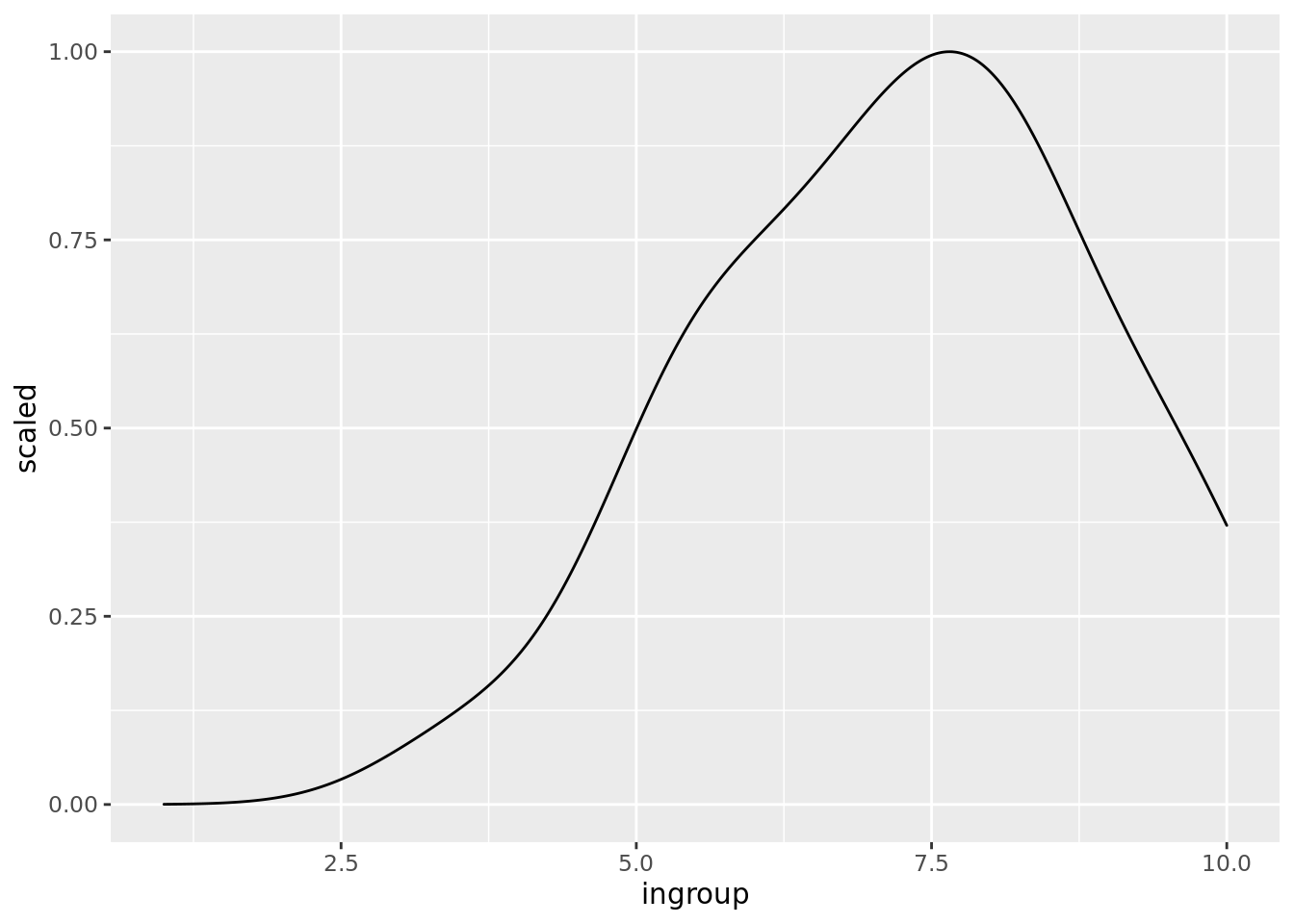In the example above, the most common (modal) rating of ingroup closeness is between 7 and 8. So, on average, people rated the ingroup closeness as quite high. However, there were quite a range of ratings, both above and below this modal rating. Your data may be different.

We can ask the same question about outgroup distance. Did everyone give bascially the same rating, or did outgroup distance vary a lot between groups? Changing `ingroup` to `outgroup` in the above command gives us the answer.

``gdata %>% ggplot(aes(outgroup)) + geom_density(aes(y=..scaled..)) + xlim(1, 10)``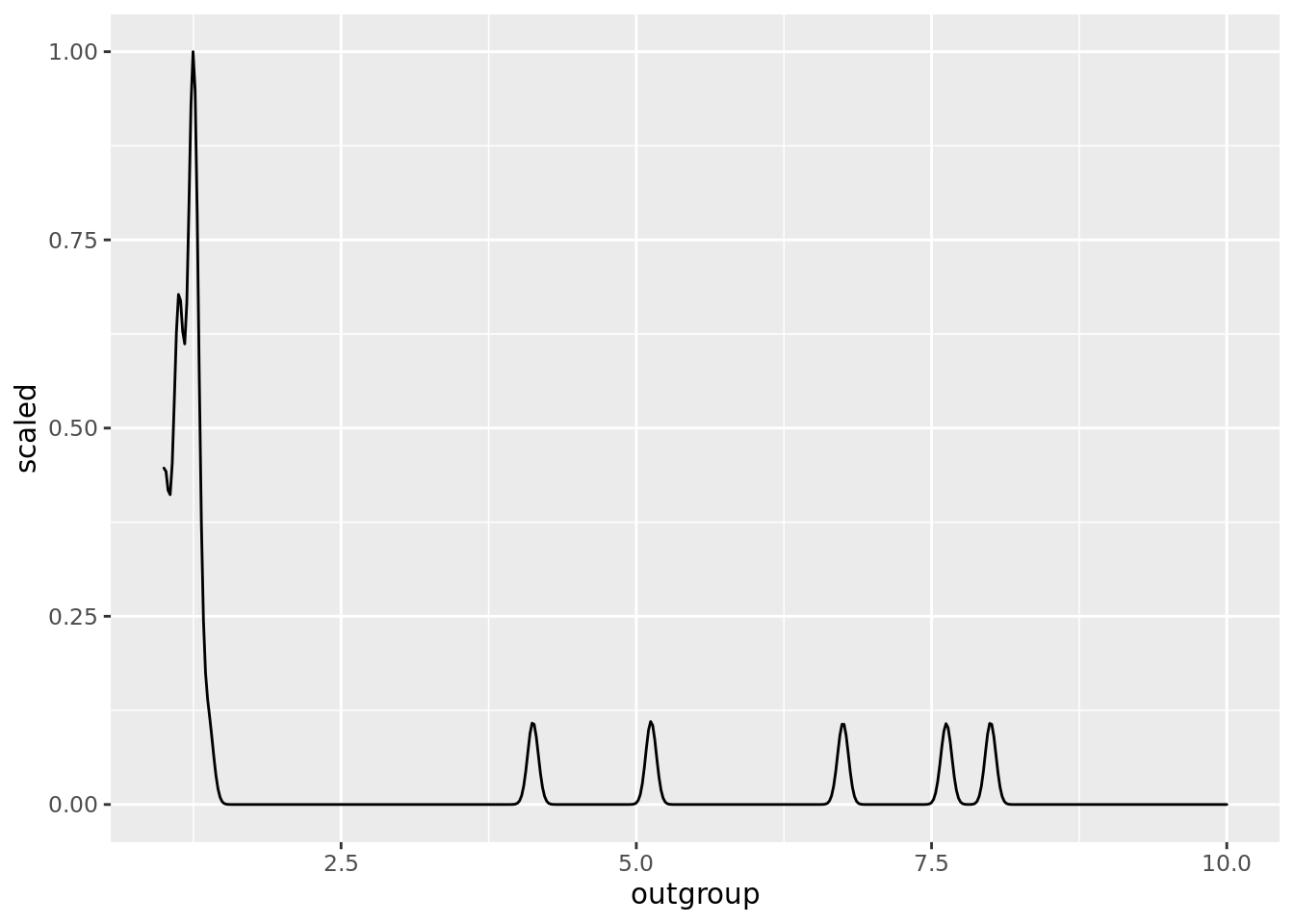In the example above, most groups gave close to the lowest possible rating (1), so we see a large peak in the plot at around 1. We also see a series of much smaller peaks, indicating that a few groups gave much higher ratings. It is possible that these mostly low ratings are due to social desirability bias – the phenomenon that people are reluctant to give answers that their social group would view negatively.

As in the last example, your data may look different.

## Scatter plots and correlation

Relevant worksheet: Face recognition

So, ingroup closeness varies between groups, as does outgroup distance (at least to some extent). Are these two sorts of variability related? For example, does high ingroup closeness tend to be associated with high outgroup distance – perhaps feeling close to your ingroup is associated with feeling distant from your outgroup?

Or perhaps high ingroup closeness is associated with low outgroup distance — feeling close to your own group also makes you feel close to other groups? Or, a third option, perhaps the two things are unrelated — whether you have high or low ingroup closeness does not predict your outgroup distance.

One way to look at this question is to produce a scatterplot. On a scatterplot, each point represents one group. That point’s position on the x-axis represents their ingroup closeness, and that point’s position on the y-axis represents their outgroup distance.

The command to produce a scatterplot in R is much like the command for a bar graph, as you used in, for example, the face recognition worksheet. The only difference is that we use the `geom_point()` command (because the graph is a set of dots or points) rather than the `geom_col()` command we used for bar (column) charts.

``gdata %>% ggplot(aes(x = ingroup, y = outgroup)) + geom_point() ``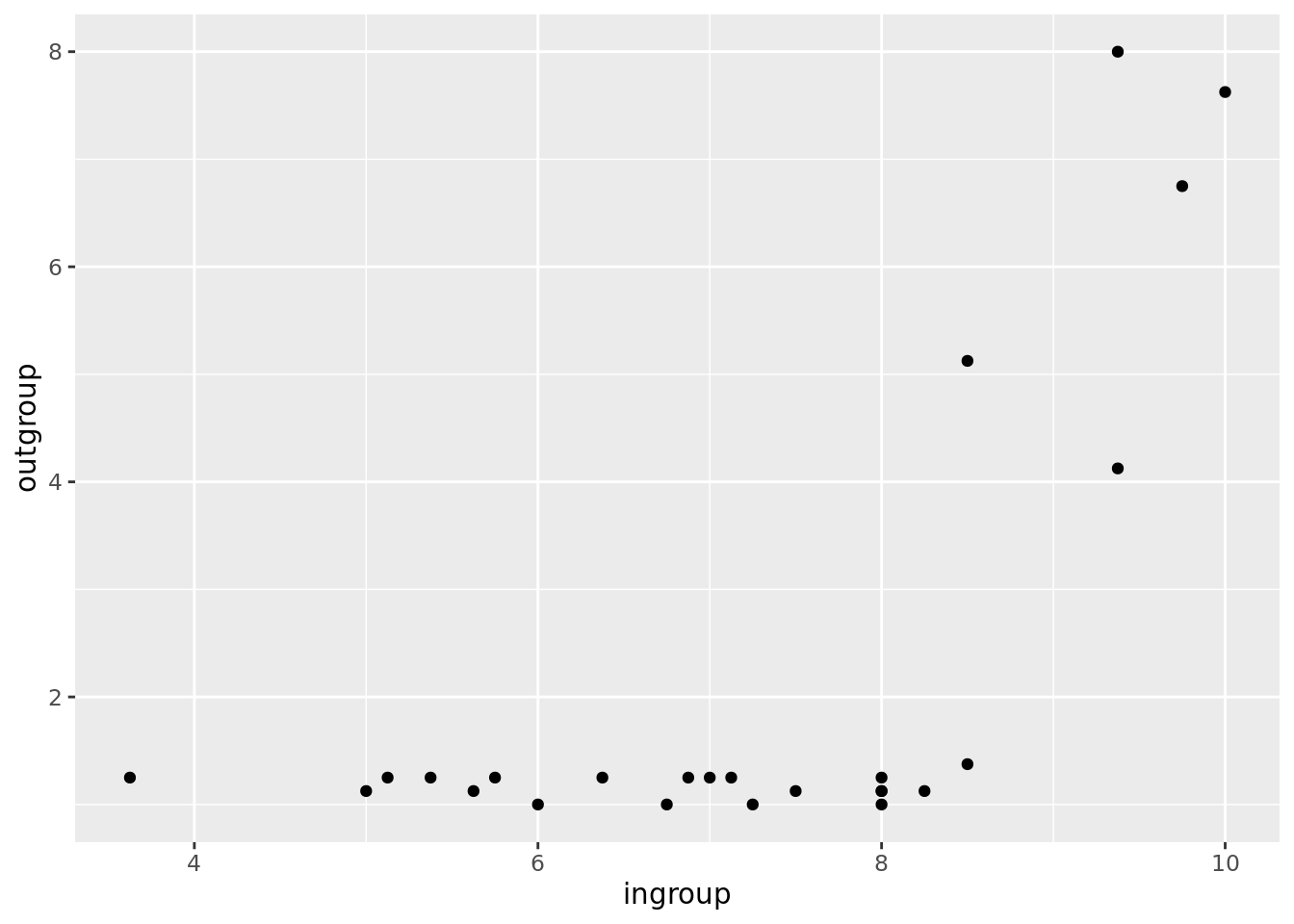In the above example, many of the points are close to the x axis. This is becasue, as we saw above, most groups gave a rating close to 1 for outgroup distance. However, once we get to an ingroup closeness above 8, an interesting pattern starts to emerge. As ingroup closeness increases from 8 to 10, outgroup distance rises from around 1 to around 7 or 8.

So it seems that, in this example dataset, ingroup closeness and outgroup distance are related. We call this type of relationship a correlation.

## Measuring correlation

Relevant worksheets: Group differences

Sometimes, it’s useful to have a single number that summarises how well two variables are correlated. We can calculate this number, called a correlation co-efficient, using the `cor` command in R.

``cor(gdata\$ingroup, gdata\$outgroup)``
`` 0.6641777``

### Explanation of command

The command is used in a similar way to the `cohen.d` command you used to calculate effect size in the group differences worksheet:

`cor()` - The command to calculate a correlation co-efficient.

`gdata\$ingroup` - One variable is in the `ingroup` column of the `gdata` data frame.

`,` - this comma needs to be here so R knows where one variable ends and the other begins.

`gdata\$outgroup` - The other variable is in the `outgroup` column of the `gdata` data frame.

### Explanation of output

In the above example, the correlation co-efficient was about 0.66. By tradition, we use a lower case r to represent a correlation co-efficient, so here r = 0.66. In order to make sense of this number, you need to know that the biggest r can ever be is 1, and the smallest it can ever be is -1.

Where r = 1: A correlation of 1 means a perfect linear relationship. In other words, there is a straight line you can draw that goes exactly through the centre of each dot on your scatterplot. The line can be shallow, or steep. Here are some examples: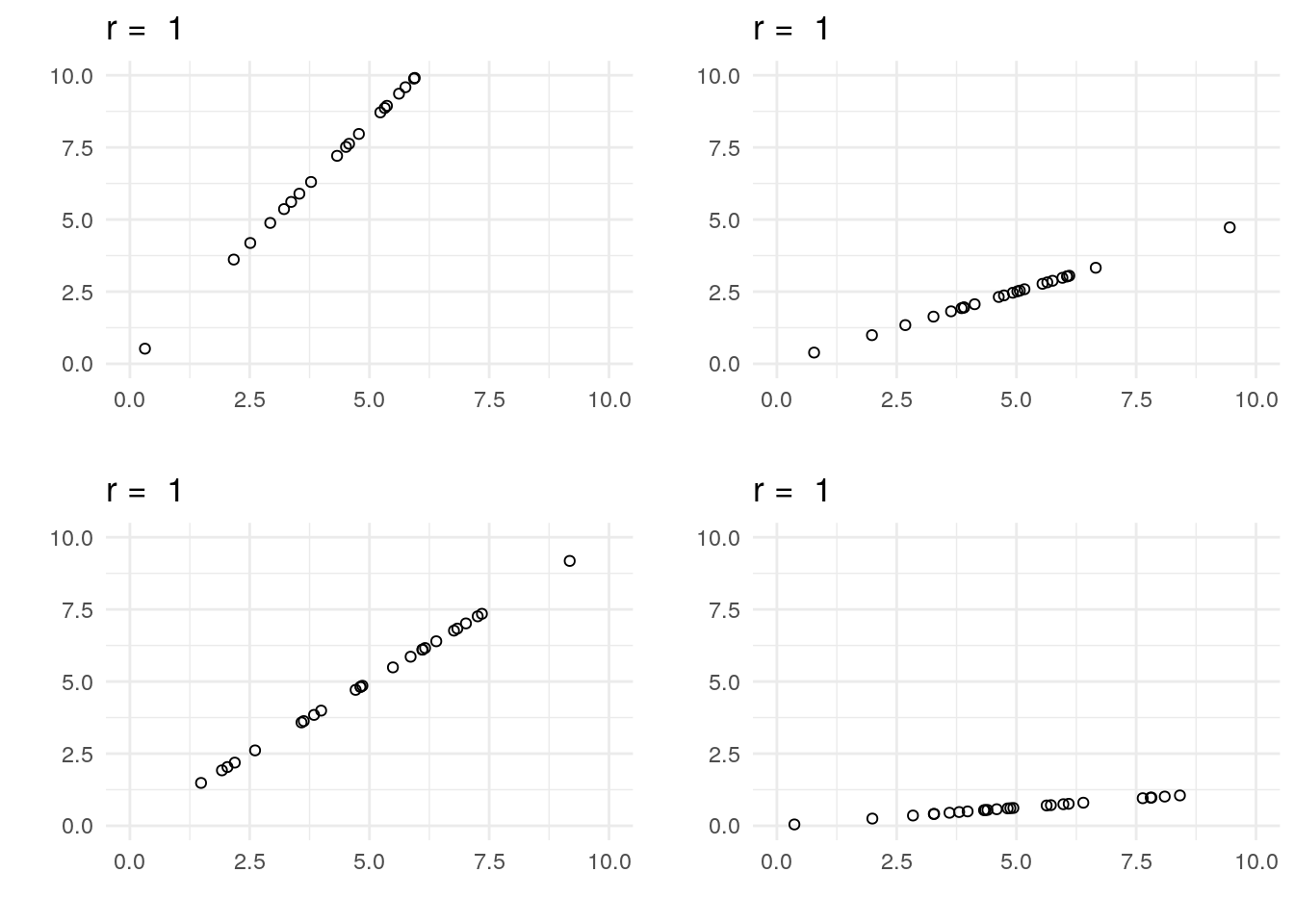Where r = 0: A correlation of zero means there is no relationship between the two variables. Here are some examples: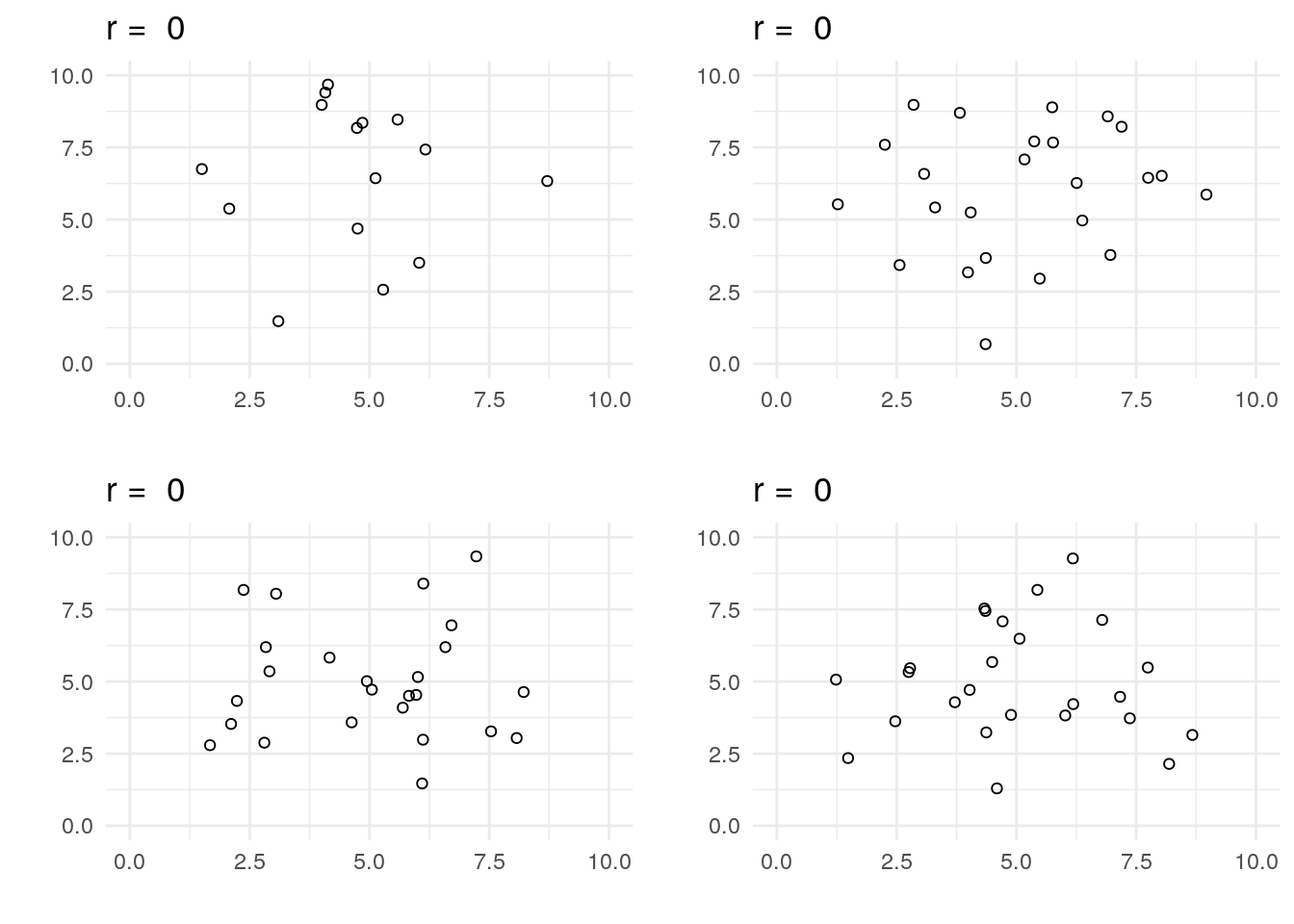Where r is between 0 and 1: As the correlation co-efficient gets further from zero, the relationship between the two variables becomes more like a straight line. Here are some more examples: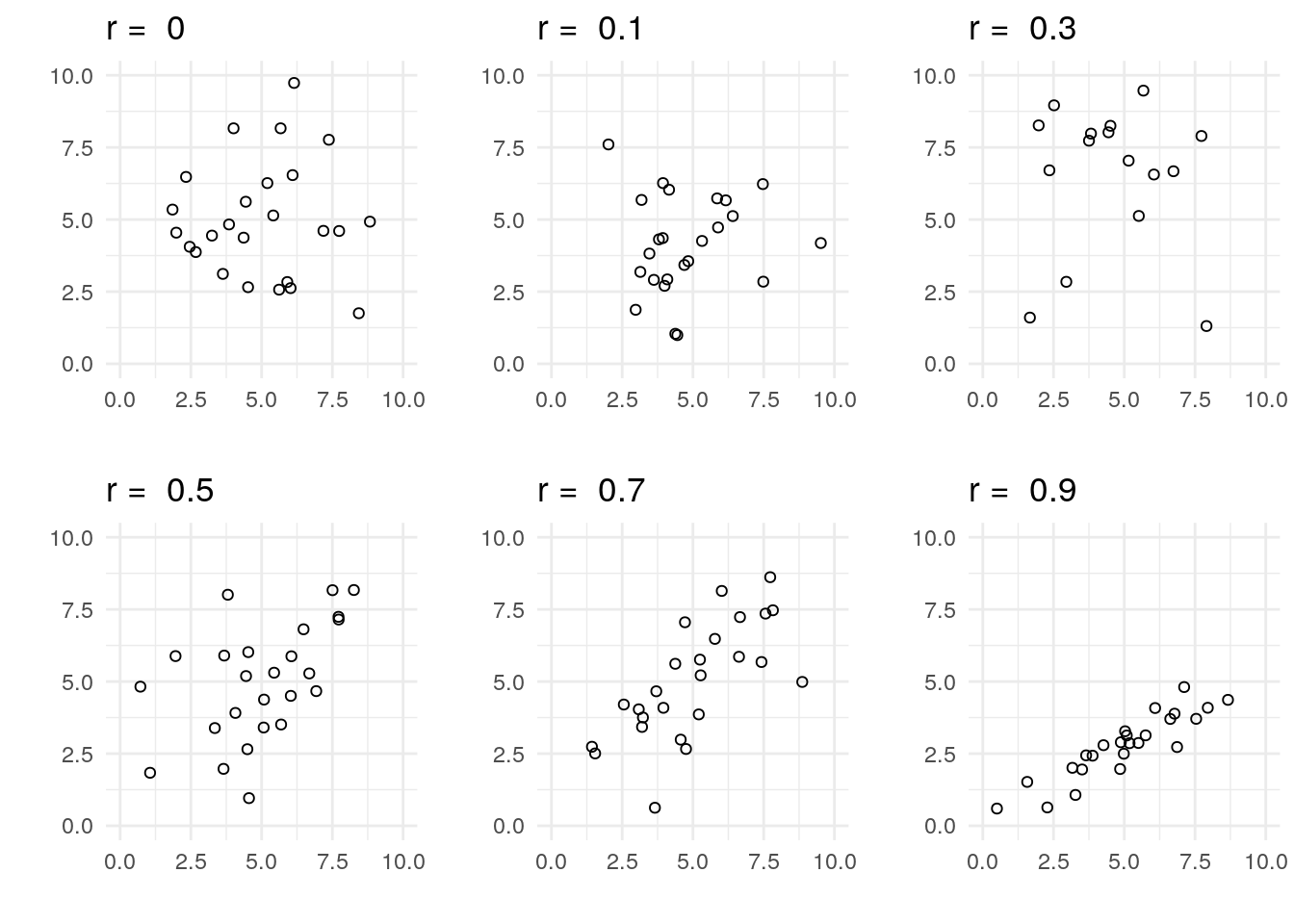Where r is less than 0: A negative correlation co-efficient just means that, as one variable gets larger, the other gets smaller: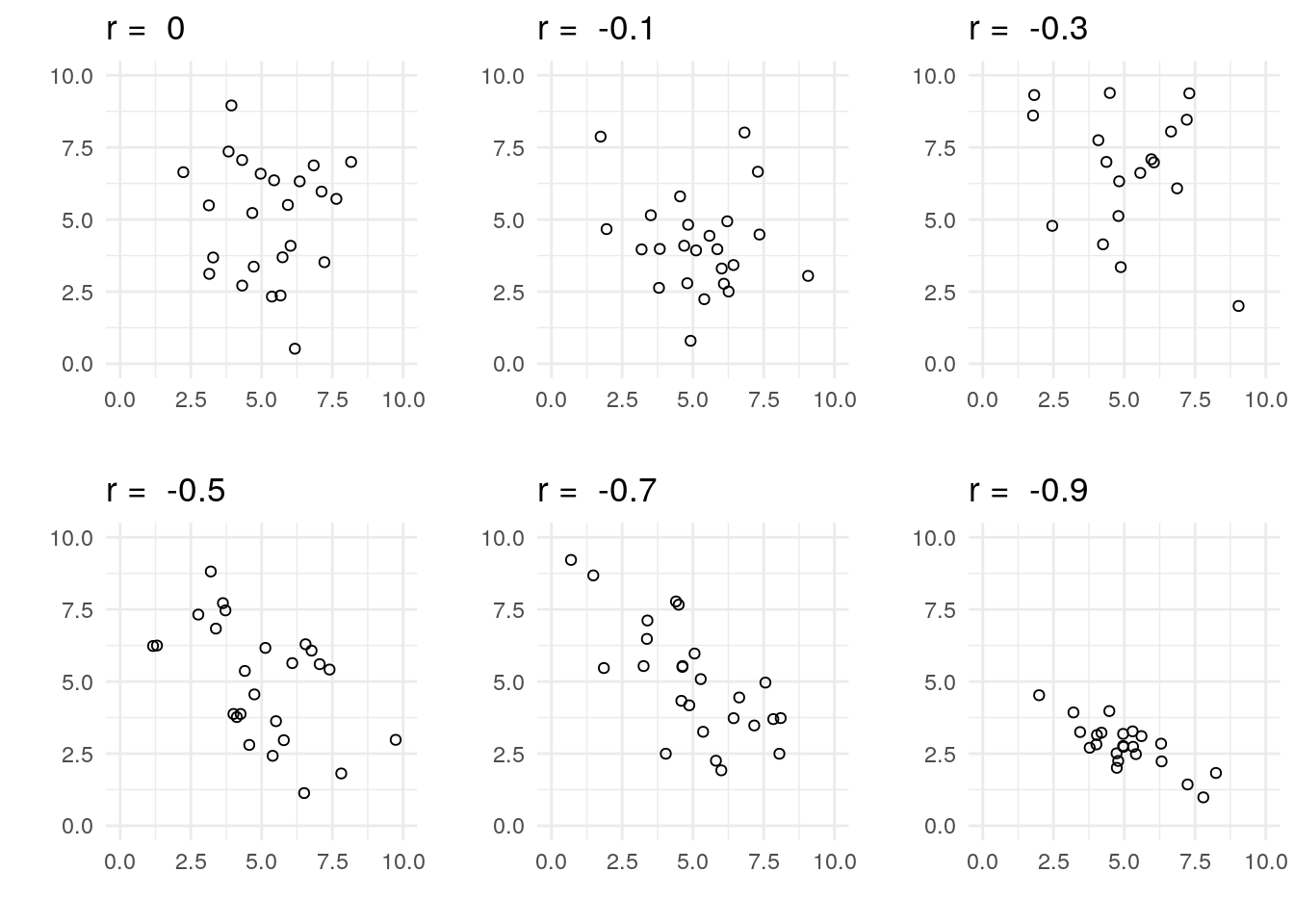### Interpreting correlation co-efficients

Relevant worksheets: Group differences

A correlation co-efficient is much like an effect size, which we covered in the group differences worksheet. More specifically, it measures the strength of the relationship between the two variables (sometimes called the covariance), relative to the variance of each variable considered on its own.

Jacob Cohen suggested the following conventions in describing correlation co-efficients: a co-efficient of 0.1 is described as a weak relationship, a correlation of 0.3 is described as a moderate association, and a correlation of 0.5 is described as a strong relationship. Not all psychologists agree with these descriptions.

## Evidence for correlation

Relevant worksheet: Evidence

So far, we’ve produced a scatterplot of ingroup closeness versus outgroup distance, and we’ve calculated a correlation co-efficient for that relationship ( r=0.66 in the example above ). But is the relationship between these two variables real, or a fluke? Much like the Bayesian t-test we calculated in the evidence worksheet, we can calculate a Bayes Factor for the relationship between two variables.

The first step is to load the BayesFactor packages, which we previously used in the evidence worksheet.

``library(BayesFactor, quietly = TRUE)``

Then, we use the `correlationBF` command, which has a similar format to the `cor` command above.

``correlationBF(gdata\$ingroup, gdata\$outgroup)``
``````Bayes factor analysis
--------------
 Alt., r=0.333 : 89.70525 ±0%

Against denominator:
Null, rho = 0
---
Bayes factor type: BFcorrelation, Jeffreys-beta*``````

The Bayes Factor is reported on the third line, towards the right. In this example, our Bayes Factor is about 89.7. This means it’s about ninety times as likely there is a relationship between these two variables as there isn’t. This is larger than the conventional threshold of 3, so psychologists will generally believe you when you claim that there is a relationship between ingroup closeness and outgroup distance. If the Bayes Factor had been less than 0.33, this would have been evidence that there was no relationship.

As we covered in the Evidence worksheet, psychologists have typically reported p values, despite the fact that p values are widely misinterpreted. If you want to calculate a p value for a correlation co-efficient, you can use the following command.

``cor.test(gdata\$ingroup, gdata\$outgroup)``
``````
Pearson's product-moment correlation

data:  gdata\$ingroup and gdata\$outgroup
t = 4.2608, df = 23, p-value = 0.0002939
alternative hypothesis: true correlation is not equal to 0
95 percent confidence interval:
0.3647781 0.8390981
sample estimates:
cor
0.6641777 ``````

The p value in this case is about .00029. The p value is not the probability that the null hypothesis is false, nor is it anything else that is both clear and useful (see the Evidence worksheet for more details). However, the value of .00029 is lower than the conventional .05 cutoff. This means psychologists will generally believe you when you claim that there is a relationship between ingroup closeness and outgroup distance.